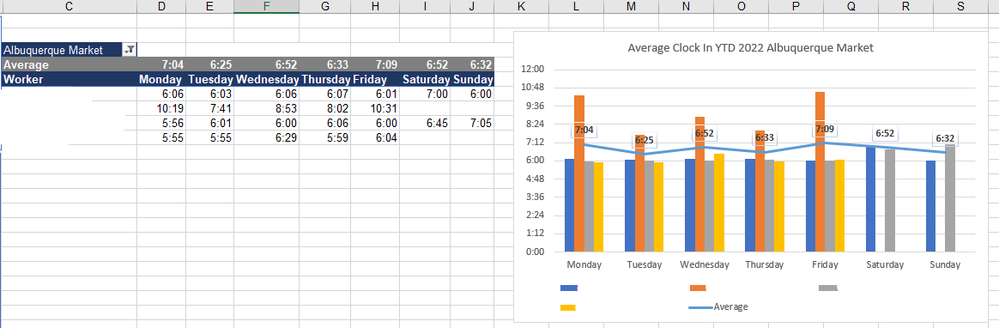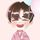cancel
Showing results for
Did you mean:Regular Visitor

## Time Stamp Analysis Visual

Hi everyone! I am doing an HR analysis on "average time workers log in by day of the week" and I need a visual of it as well. I tried converting the time stamp (HHMM) to numeric value but it just doesn't represent the desired time format on the visual. Below is the mockup I did on Excel. Would love to rPlease help! Thank you!1 ACCEPTED SOLUTIONCommunity Support

Hi @JodyLin ,

To my knowledge, your requirement——Show Time format value for Y-axis could not be realized currently.

Support y-axis time values

Chart Time HH:MM:SS in Y-Axis

dates and times on the y-axis of a graph

My workaround is Switch Time to Number type for Y-axis , and apply the Time format to Tooltips pane:

1. Add column: get total seconds

``Time(Seconds) = HOUR([Time]) *3600 +MINUTE([Time])*60+SECOND([Time])``

2. Create a measure to get the average seconds, and then change to Time type

``````Avg Time format =
var avgSec= CALCULATE(AVERAGE('Table'[Time(Seconds)]),ALLEXCEPT('Table','Table'[WeekDay]))
var h=INT(avgSec/3600)
var m=INT( (avgSec - h*3600) / 60)
var s=avgSec-  h*3600 -m*60
return  TIME(h,m,s)``````

Output:Best Regards,
Eyelyn Qin
If this post helps, then please consider Accept it as the solution to help the other members find it more quickly.

2 REPLIES 2Community Support

Hi @JodyLin ,

To my knowledge, your requirement——Show Time format value for Y-axis could not be realized currently.

Support y-axis time values

Chart Time HH:MM:SS in Y-Axis

dates and times on the y-axis of a graph

My workaround is Switch Time to Number type for Y-axis , and apply the Time format to Tooltips pane:

1. Add column: get total seconds

``Time(Seconds) = HOUR([Time]) *3600 +MINUTE([Time])*60+SECOND([Time])``

2. Create a measure to get the average seconds, and then change to Time type

``````Avg Time format =
var avgSec= CALCULATE(AVERAGE('Table'[Time(Seconds)]),ALLEXCEPT('Table','Table'[WeekDay]))
var h=INT(avgSec/3600)
var m=INT( (avgSec - h*3600) / 60)
var s=avgSec-  h*3600 -m*60
return  TIME(h,m,s)``````

Output:Best Regards,
Eyelyn Qin
If this post helps, then please consider Accept it as the solution to help the other members find it more quickly.Super User
Time field only go upto 24hrs,
So convert it to decimal is you need to do aggegation
then use this dax measure to display the answer as  formated text

Time HH:MM:SS =
-- convert decimal duration to hh:mm:ss text eg converts 5.5 to "00:05:30" and convert 0 to 00:00

-- Take decimal minutes as input eg 5.5 minutes  of  a measure like  SUM(youttable[duration])
VAR DecimalMinutes = 5.5

-- Convert decimal minutes to seconds
VAR Duration = DecimalMinutes * 60

-- Calc the days, hours, minutes and seconds (add days if required
VAR Hours = INT(DIVIDE(Duration,3600,0))
VAR Minutes = INT(DIVIDE(MOD( Duration - ( Hours * 3600 ),3600),60,0))
VAR Seconds = ROUNDUP(MOD ( MOD( Duration - ( Hours * 3600 ),3600 ), 60 ),0)

-- Format hours, minutes and seconds to two decimals
VAR H = IF (LEN ( Hours ) = 1, CONCATENATE ( "0", Hours ), CONCATENATE ( "", Hours ))
VAR M = IF (LEN ( Minutes ) = 1, CONCATENATE ( "0", Minutes ), CONCATENATE ( "", Minutes ))
VAR S = IF (LEN ( Seconds ) = 1, CONCATENATE ( "0", Seconds ), CONCATENATE ( "", Seconds ))

RETURN
-- if input is 0 return 00:00.
-- return hours:mins:seconds as text
-- handles any errors(unlikely)
-- if inpout is greater than 24hrs then the hours will be displayed
IFERROR(
IF (DecimalMinutes>0,
H & ":" & M & ":" & S,
"0:00"),
"Error")

Thanks for reaching out for help.

Remeber we are unpaid volunteers.

Click the thumbs up and accept as solution button.

One question per ticket please. If you need to extend your request then please raise a new ticket.

You will get a quicker response and each volunteer solver will get the kudos they deserve. Thank you !